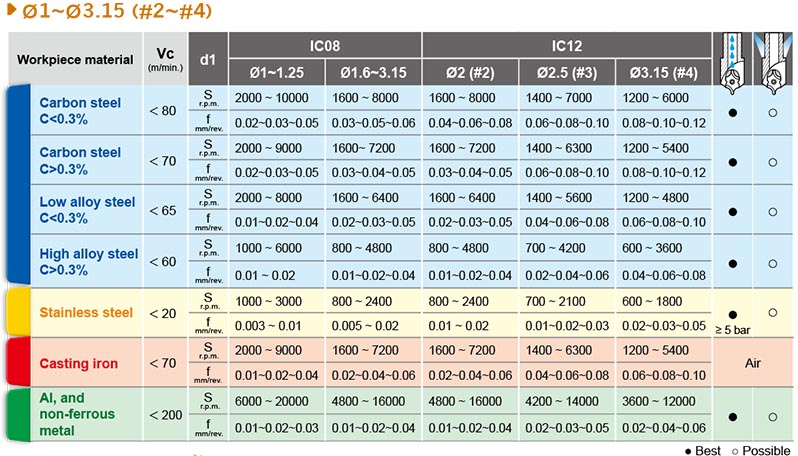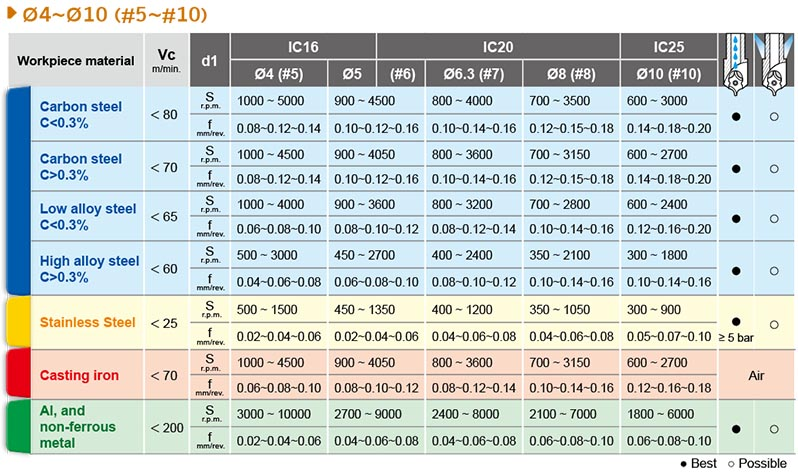• Home
• Cutting Formula of i-Center

# Cutting Formula of i-Center

i-center
● Attention of Form A+B insert:
Reduce 30% of Spindle speed and keep same feed rate (mm/rev.) while depth L2 is reached.
● Using your “d1” value and cutting speed Vc from the data sheet, calculate spindle speed “S”(r.p.m).
● “ F” feed rate per minute F = S x f = IPR x r.p.m.

Metric Formula
d1 Center diameter mm
Vc Cutting Speed m/min.
S Spindle speed r.p.m (Vc x 1000)/(π x d1)
f - mm/rev.
F - mm/min. Sxf
Inch Formula
d1 Center diameter inch
S Spindle speed r.p.m  (3.82 x SFM)/(d1)
SFM Surface speed ft./min. Vc (m/min.) x 3.28
f IPR inch/rev.
F - inch/min. IPR x r.p.m.Cutting Data